Main Content

# ISM Band Patch Microstrip Antennas and Mutually Coupled Patches

This example shows how to design and implement a rectangular, circular, triangular and elliptical patch microstrip antennas complying with the ISM (Industrial Scientific and Medical) band.

### Define Parameters

All these microstrip antennas consisting of PCB with 6.6 mm thickness with dielectric constant EpsilionR 4.2, and loss-tangent of 0.02, and square ground plane of 100 mm x 100 mm, fed by 1.3 mm diameter coaxial probe, are designed to comply with the ISM band (2.4 - 2.5 GHz).

```LGp = 100e-3; % Ground plane length WGp = 100e-3; % Ground plane width h = 6.6e-3; % Height of the substrate```

### Define Parameters Of Elliptical Patch Antenna

Design a probe feed` patchMicrostripElliptical `antenna using a dimension of 33.5 mm major axis, 18.8 mm minor axis. The feed is offset by 11.6 mm from the origin along the X-axis.

```a = 33.5e-3; % Major Axis b = 18.8e-3; % Minor Axis f = 11.6e-3; % Feed Offset d = dielectric('EpsilonR',4.2,'LossTangent',0.02);```

Create a `patchMicrostripElliptical` antenna using the defined parameters.

```p_Ellipse= patchMicrostripElliptical('MajorAxis',a,'MinorAxis',b,... 'Height',h,'Substrate',d,'GroundPlaneLength',LGp,'GroundPlaneWidth',... WGp,'FeedOffset',[-(a/2-f) 0]); figure; show(p_Ellipse);```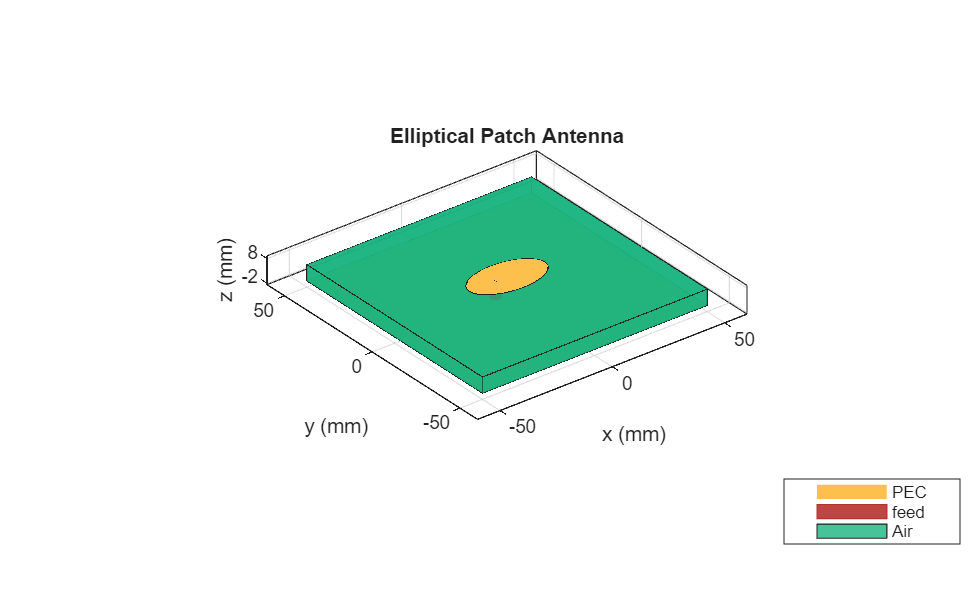### Define Parameters Of Circular Patch Antenna

Design a probe feed `patchMicrostripCircular` antenna using a dimension of 16 mm Radius. The feed is offset by 9.25 mm from the origin along the X-axis.

```r = 16e-3; % Radius f1 = 9.25e-3; % Feed Offset```

Create a `patchMicrostripCircular` antenna using the defined parameters.

```p_Circle= patchMicrostripCircular('Radius',r,'Height',h,'Substrate',d,... 'GroundPlaneLength',LGp,'GroundPlaneWidth',WGp,'FeedOffset',[-(r-f1) 0]); figure; show(p_Circle);```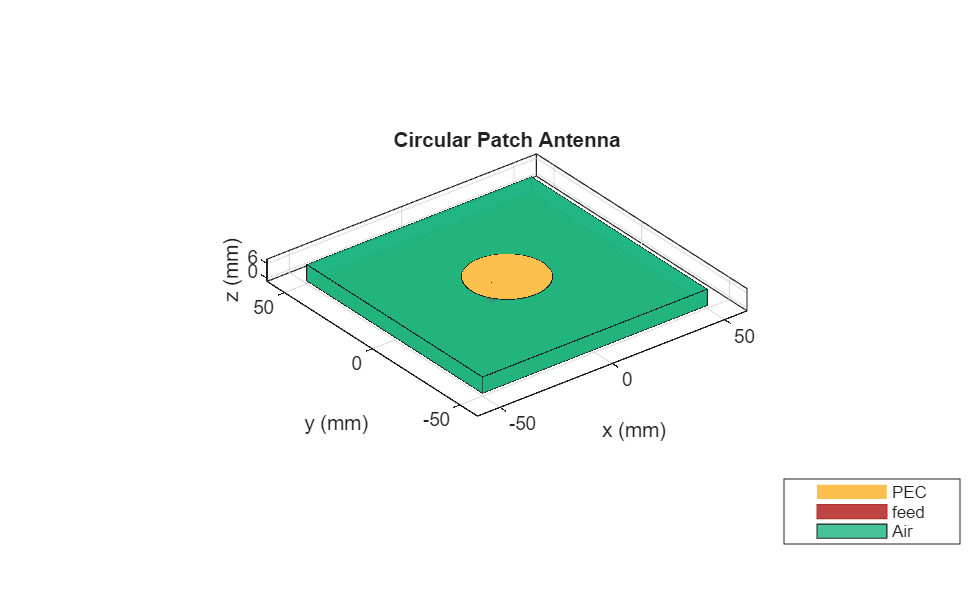### Define Parameters Of Rectangular Patch Antenna

Design a probe-fed rectangular` patchMicrostrip` antenna using a dimension of 28.20 mm length, 34.06 mm width. The feed is offset by 5.3 mm from the origin along the X-axis.

```rect1 = 28.20e-3; % length rect2 = 34.06e-3; % width f2 = 5.3e-3; % feedoffset```

Create a` patchMicrostrip` rectangular antenna using the defined parameters.

```p_rect= patchMicrostrip('Length',rect1,'Width',rect2,'Height',h,'Substrate',d,... 'GroundPlaneLength',LGp,'GroundPlaneWidth',WGp,... 'FeedOffset',[-(rect1/2-f2) 0]); figure; show(p_rect);```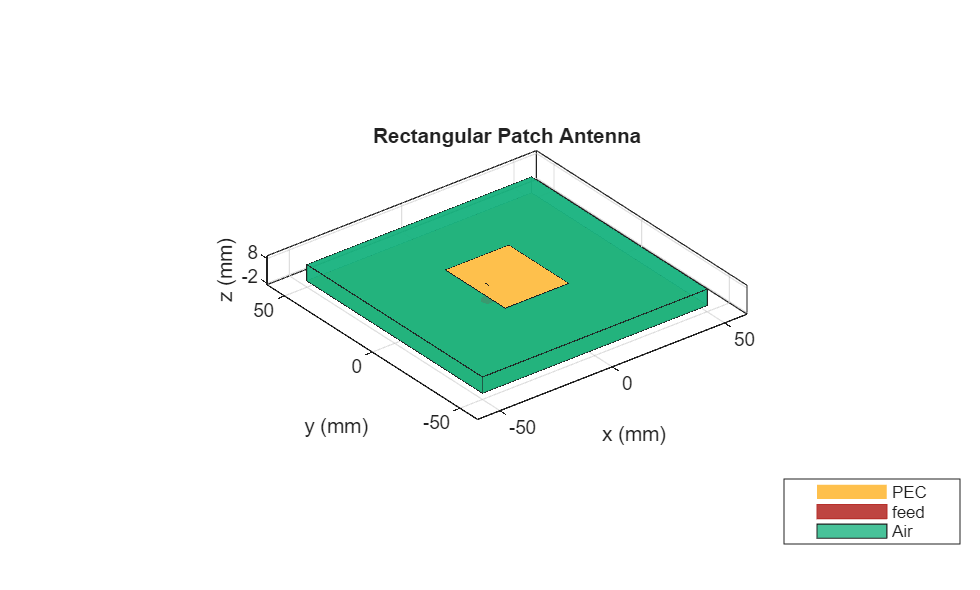### Define Parameters of Triangular Patch Antenna

Design an equilateral `patchMicrostripTriangular `antenna using a dimension of 37.63 mm side. The feed is offset by 3.8 mm from the origin along the Y-axis.

```side = 37.63e-3; f_off = 3.8e-3; p_triang= patchMicrostripTriangular('side',side,'Height',h,'Substrate',d,... 'GroundPlaneLength',LGp,'GroundPlaneWidth',WGp,... 'FeedOffset',[0 -side/2+f_off]); figure; show(p_triang);```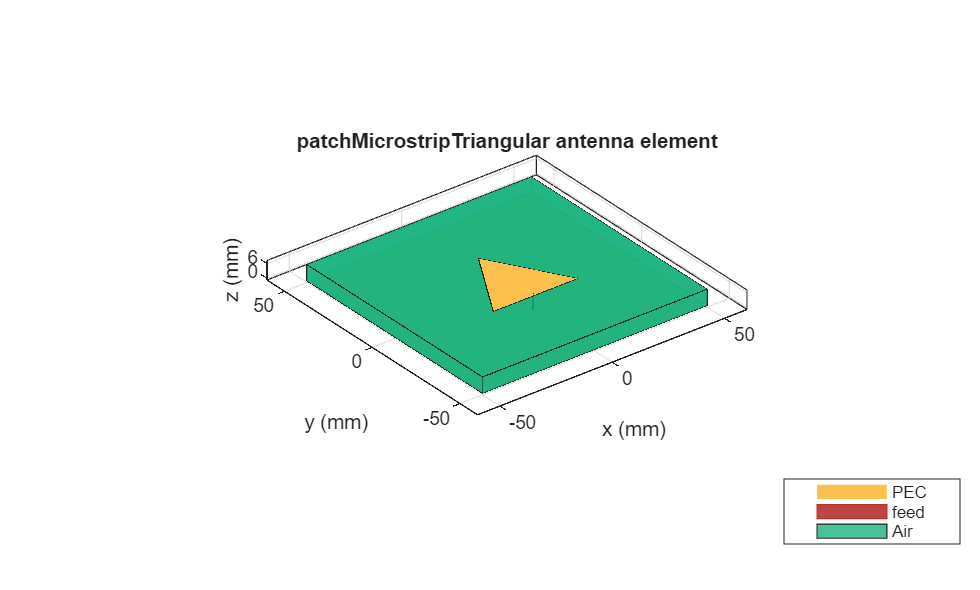### Visualize Reflection Coefficient Magnitude of Patches

Plot the reflection coefficient for these antennas over the band and a reference impedance of 50 ohms. Curves for the reflection coefficient magnitude are shown in the below figure. Manually mesh of patch antennas with different edge lengths.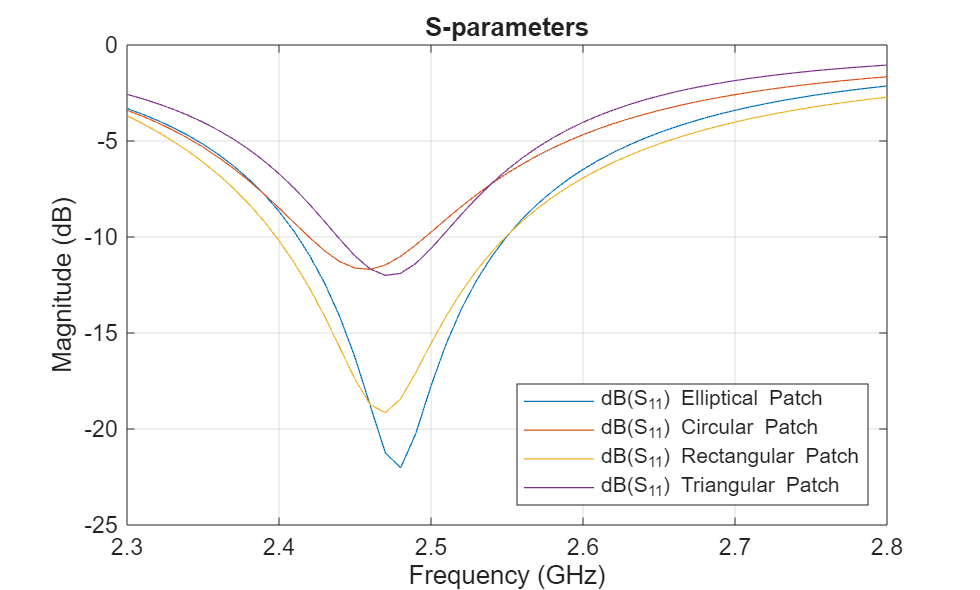### Visualize Radiation Pattern

The directivity of the antennas are around 6.37 dB for Elliptical patch, 7 dB for circular patch, 7.37 dB for rectangular patch and 6.16 dB for triangular patch.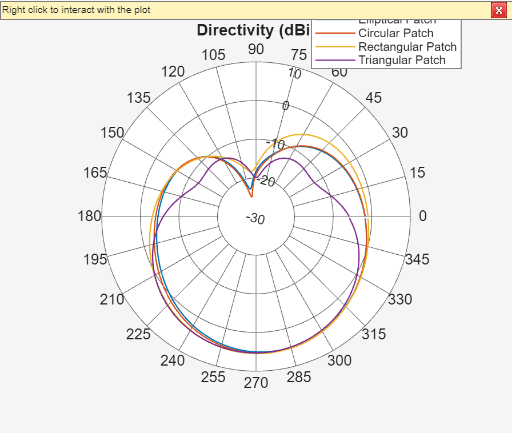### Mutually Coupling Between Rectangular and Triangular Patches

This section is devoted to the study of cases, focusing on configurations with the lowest mutual couplings. The relative displacement 'd' is fixed as lambda/2, two patches placed side-by-side configuration and the patches positioned in the center of the rectangular 160 mm x 100 mm ground plane with a substrate of FR4.

```LGp1 = 160e-3; % Ground plane length WGp1 = 100e-3; % Ground plane width Ground_plane1=antenna.Rectangle('Length',LGp1,'Width',WGp1);% Ground plane dis = 0.0612; % distance between two patches (d = lambda/2)```

Create a `patchMicrostrip` rectangular antenna using the defined parameters.

```r_ant = pcbStack(p_rect); rect_p = r_ant.Layers{1}; rect_p.Center = [-dis/2 0];```

Create a `patchMicrostripTriangular` antenna using the defined parameters.

```t_ant = pcbStack(p_triang); triangle_p = t_ant.Layers{1}; triangle_p= rotateZ(triangle_p,180); triangle_p= translate(triangle_p,[dis/2, 0, 0]); patch = rect_p+triangle_p; % adding patches```

### Define PCB Stack

Use the `pcbStack` to define the metal and dielectric layers for mutually coupled patch antenna. the top-most layer is a patch layer, the second layer is dielectric layer, and the third layer is the ground plane.

```p_mc=pcbStack; d4=dielectric('EpsilonR',4.2,'Thickness',h,'LossTangent',0.02); p_mc.BoardThickness=d4.Thickness; p_mc.BoardShape.Length=LGp1; p_mc.BoardShape.Width=WGp1; p_mc.Layers={patch,d4,Ground_plane1}; p_mc.FeedLocations=[-dis/2 -(rect1/2-f2) 1 3; dis/2 -5.3075e-3 1 3]; p_mc.FeedDiameter=1.3e-3; figure; show(p_mc);```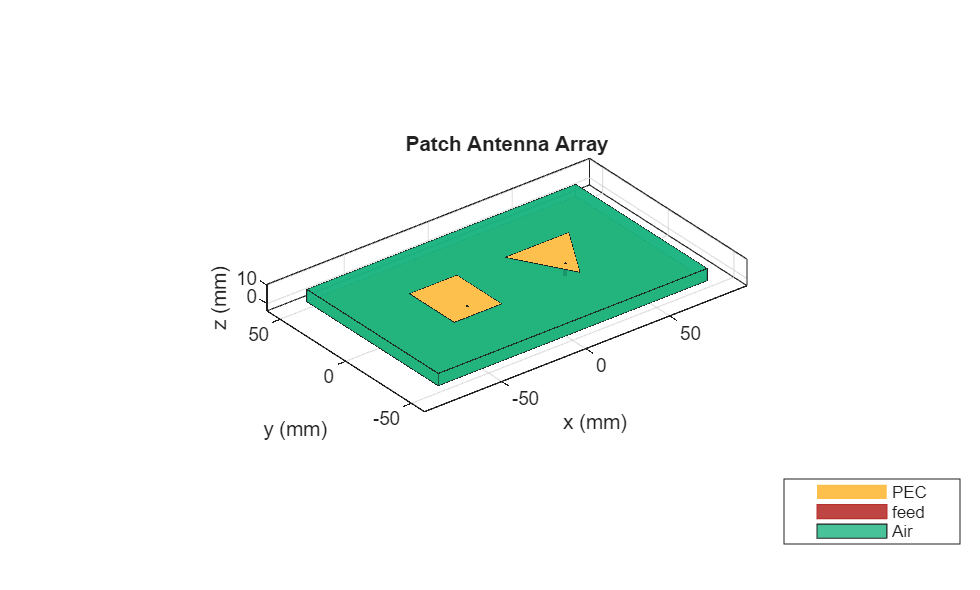### Radiation Pattern of Mutually Coupled Patches

The side-by -side configuration directivity is 7.4 dB

```figure; pattern(p_mc,2.45e9);```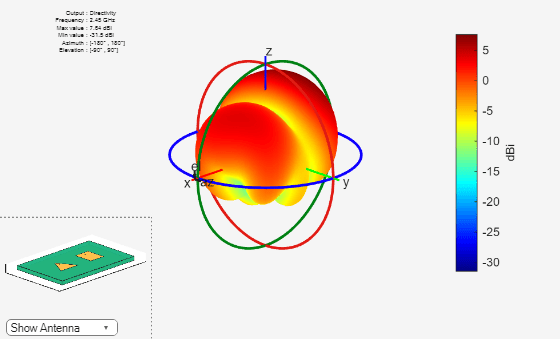### Pattern Magnitude

```figure; [fm,~,t10] = pattern(p_mc,2.45e9,0,0:360); polarpattern(t10,fm);```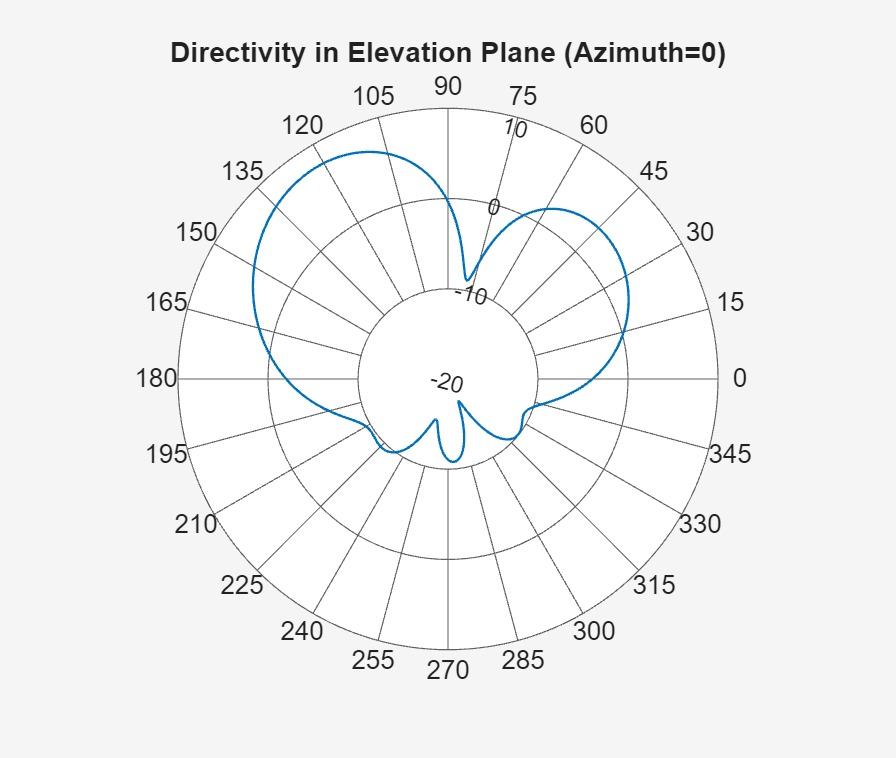### Reference

2) Probe - Fed Linearly - Polarized Electrically Equivalent Microstrip Antennas on FR4 Substrates

## Support

#### Hybrid Beamforming for Massive MIMO Phased Array Systems

Download the white paper